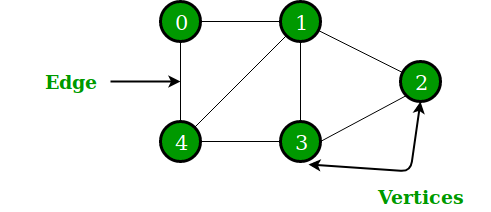# What is a graph data structure?

Graph is a type of non-linear data structure that consists of vertices or nodes connected by edges or links for storing data. Edges connecting the nodes may be directed or undirected.

A Graph is a non-linear data structure consisting of nodes and edges. The nodes are sometimes also referred to as vertices and the edges are lines or arcs that connect any two nodes in the graph. More formally a Graph can be defined as, … Graphs are also used in social networks like linkedIn, Facebook.

A graph is a non-linear data structure which is a collection of vertices (also called nodes) and edges that connect these vertices. A graph is often viewed as a generalisation of the tree structure, where instead of a purely parent-to-child relationship between tree nodes, any kind of complex relationships between the nodes can exist. In a tree structure, nodes can have any number of children but only one parent, a graph on the other hand relaxes all such kinds of restrictions.

A graph can also be used to represent a computer network where the nodes are workstations and the edges are the network connections. Graphs have so many applications in computer science and mathematics that several algorithms have been written to perform the standard graph operations, such as searching the graph and finding the shortest path between the nodes of a graph.